Ex 9.1

Chapter 9 Class 7 Perimeter and Area
Serial order wise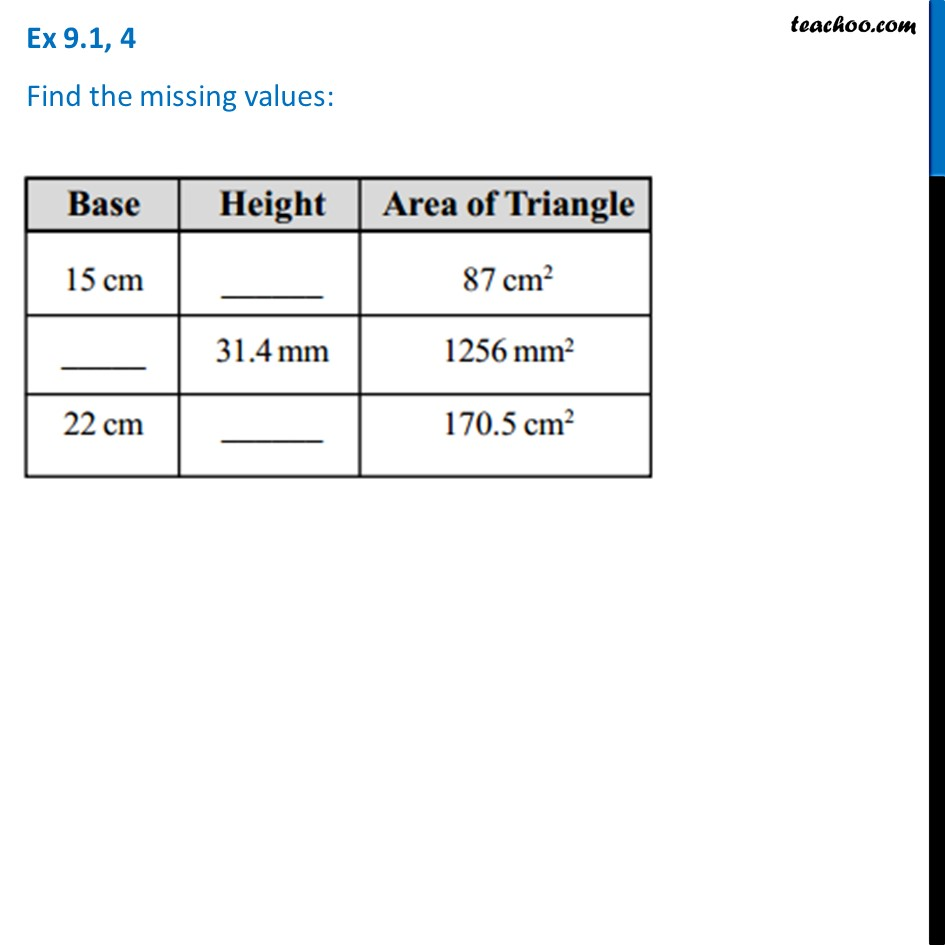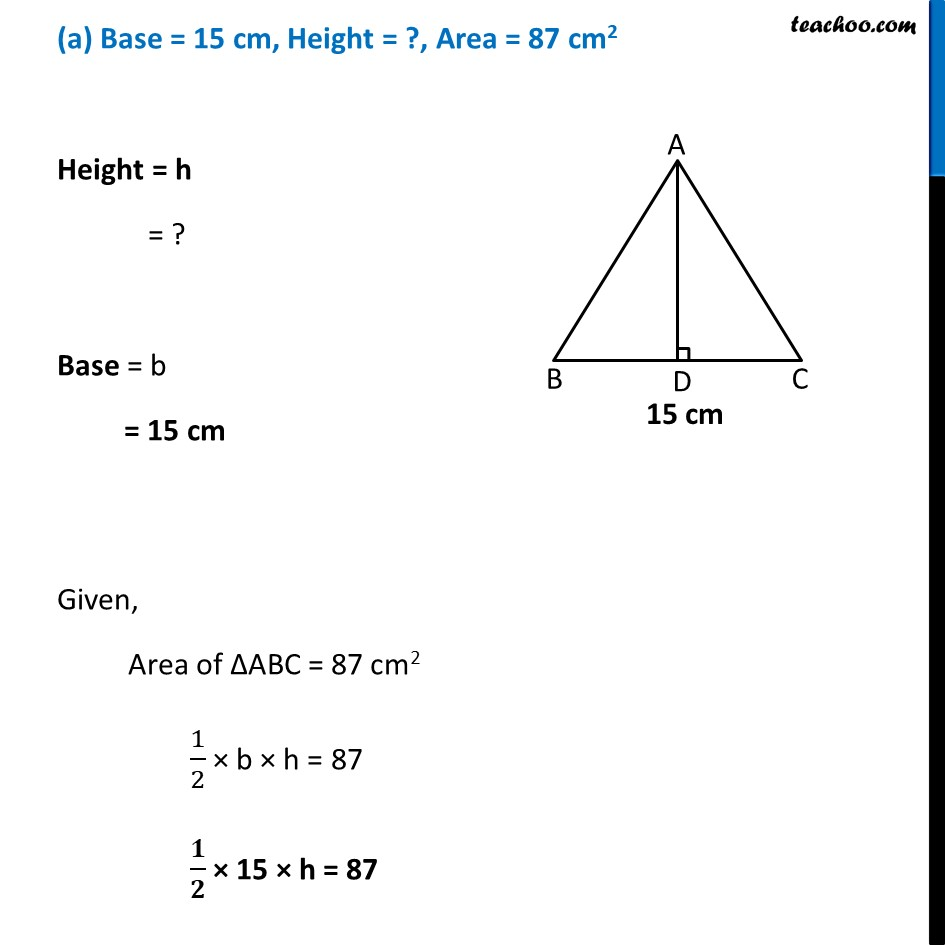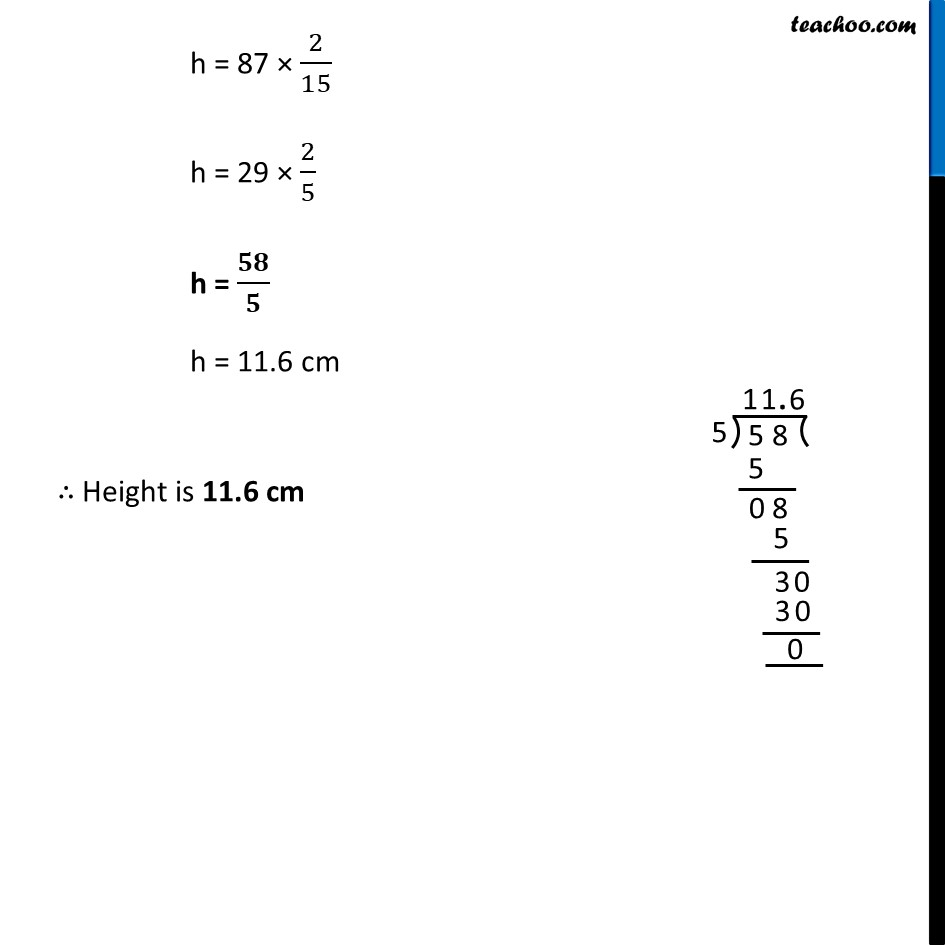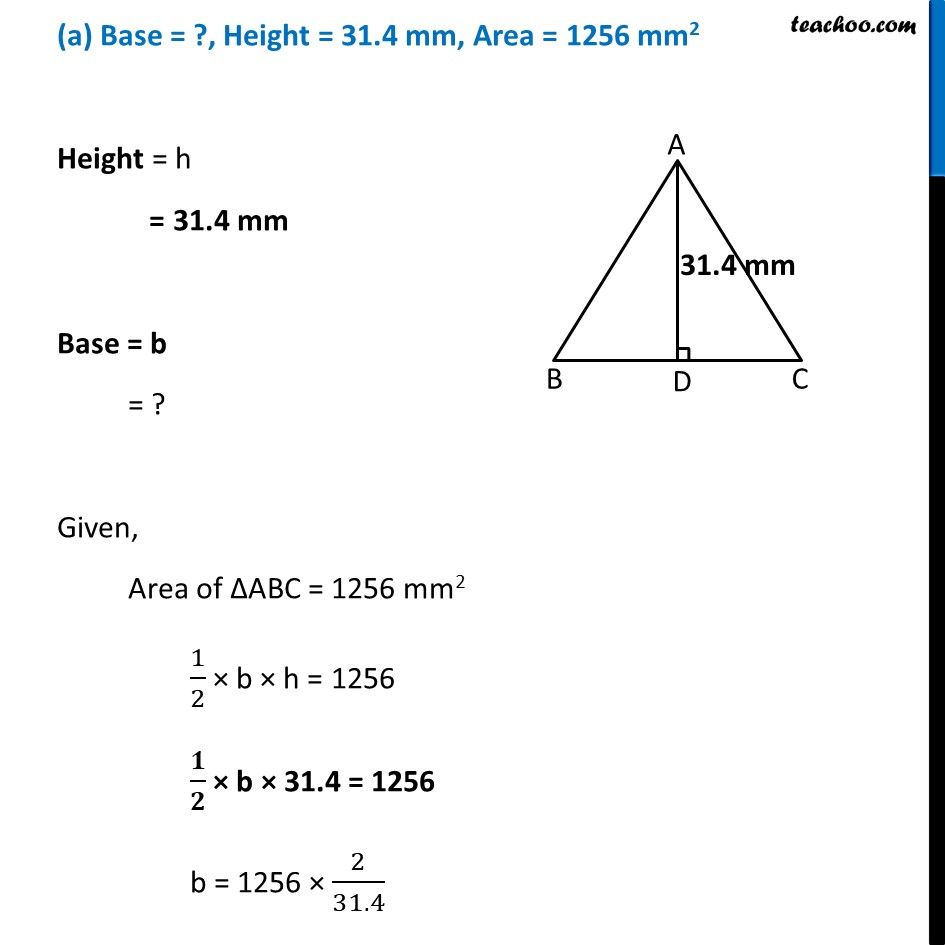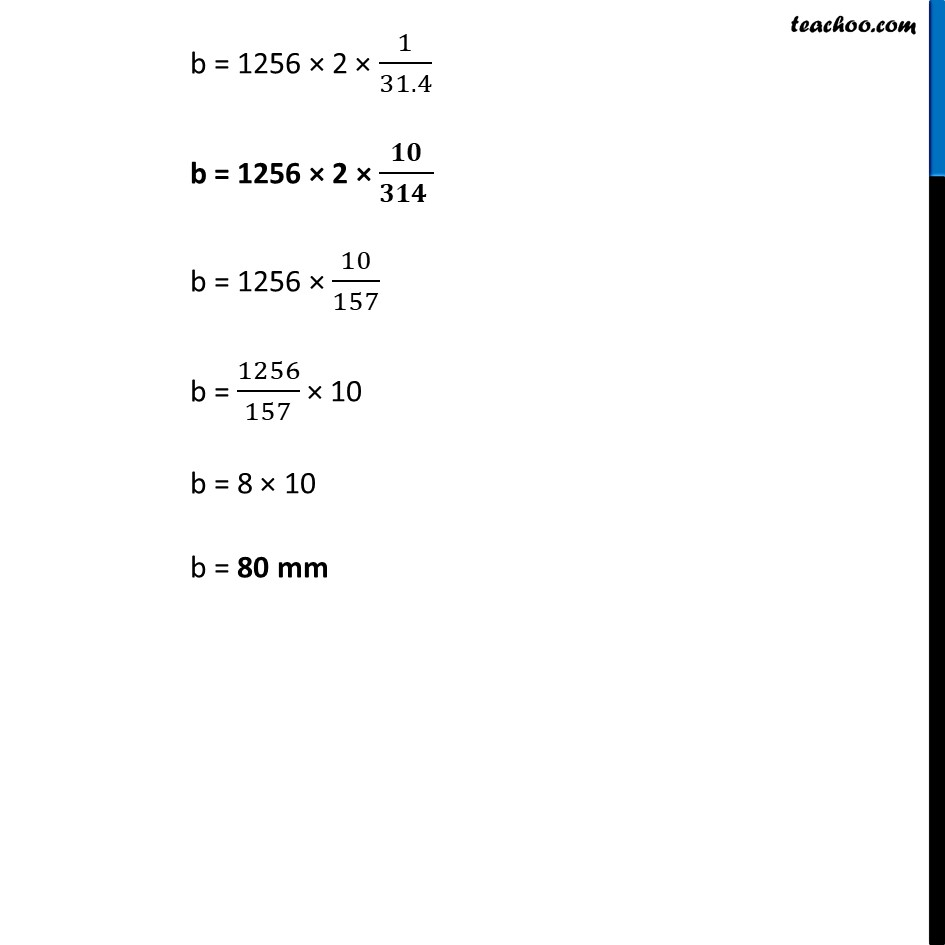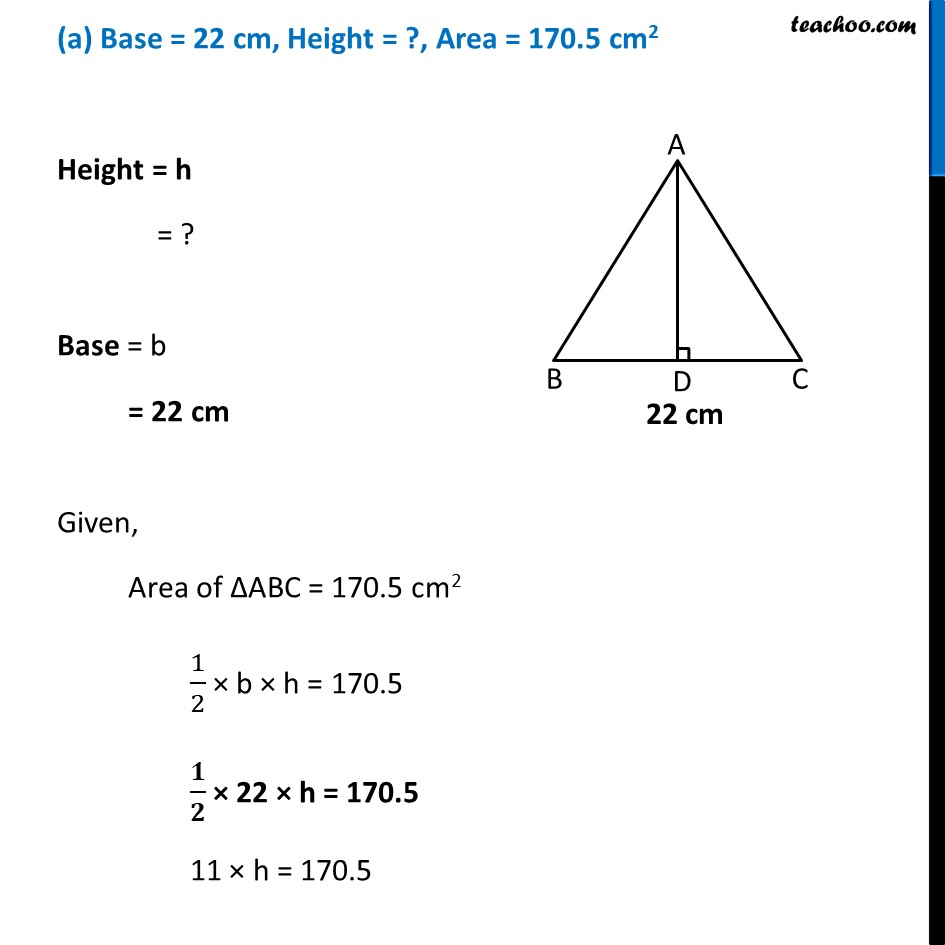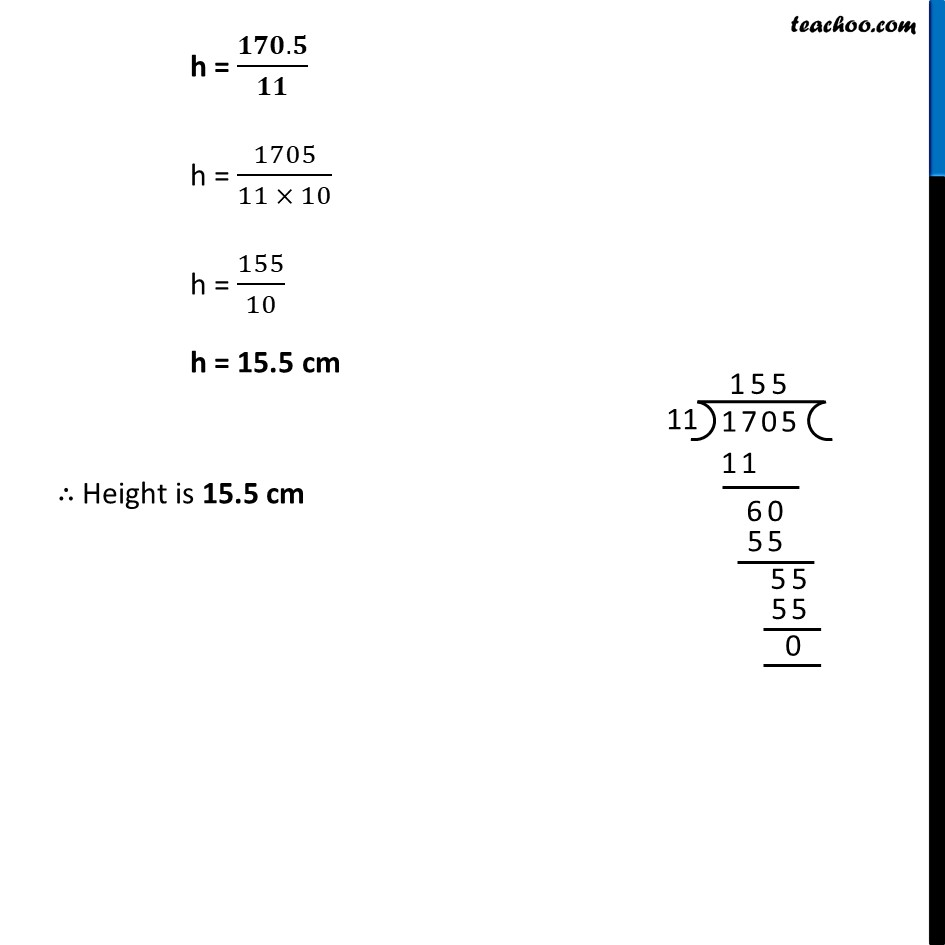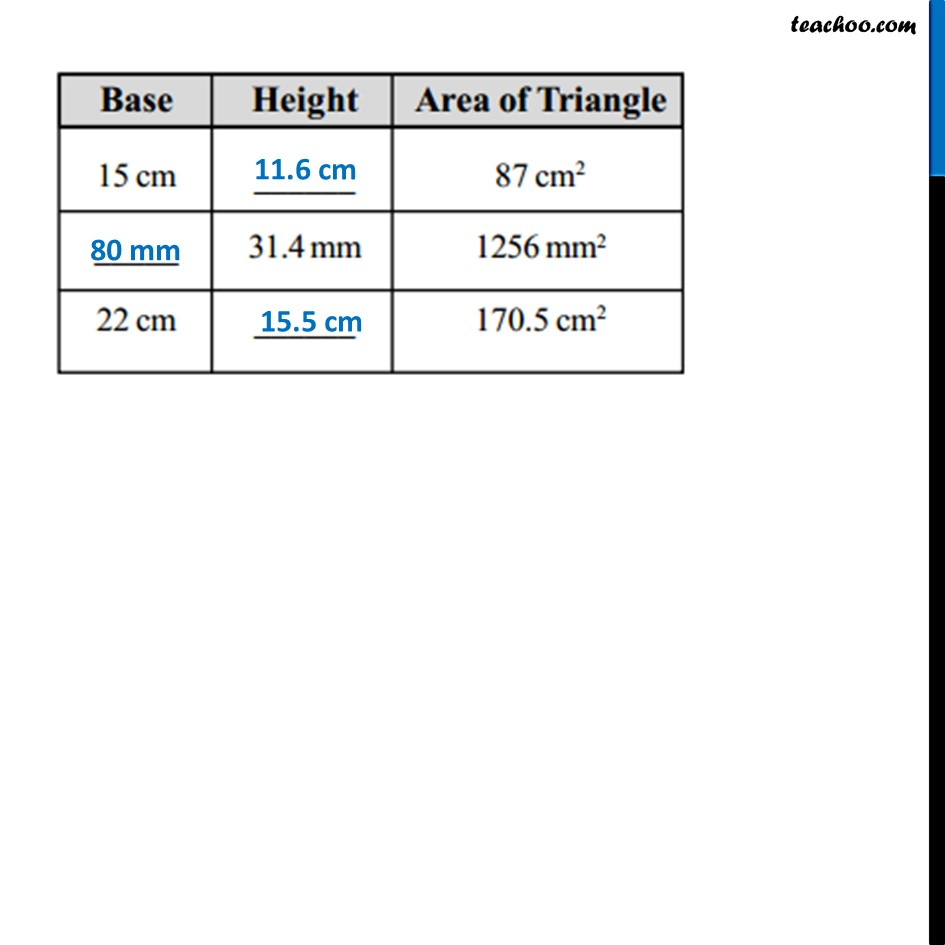Learn in your speed, with individual attention - Teachoo Maths 1-on-1 Class

### Transcript

Ex 9.1, 4 Find the missing values: (a) Base = 15 cm, Height = ?, Area = 87 cm2 Height = h = ? Base = b = 15 cm Given, Area of ∆ABC = 87 cm2 1/2 × b × h = 87 𝟏/𝟐 × 15 × h = 87 h = 87 × 2/15 h = 29 × 2/5 h = 𝟓𝟖/𝟓 h = 11.6 cm ∴ Height is 11.6 cm (a) Base = ?, Height = 31.4 mm, Area = 1256 mm2 Height = h = 31.4 mm Base = b = ? Given, Area of ∆ABC = 1256 mm2 1/2 × b × h = 1256 𝟏/𝟐 × b × 31.4 = 1256 b = 1256 × 2/31.4 b = 1256 × 2 × 1/31.4 b = 1256 × 2 × 𝟏𝟎/(𝟑𝟏𝟒 ) b = 1256 × 10/157 b = 1256/157 × 10 b = 8 × 10 b = 80 mm (a) Base = 22 cm, Height = ?, Area = 170.5 cm2 Height = h = ? Base = b = 22 cm Given, Area of ∆ABC = 170.5 cm2 1/2 × b × h = 170.5 𝟏/𝟐 × 22 × h = 170.5 11 × h = 170.5 h = (𝟏𝟕𝟎.𝟓)/𝟏𝟏 h = 1705/(11 × 10) h = 155/10 h = 15.5 cm ∴ Height is 15.5 cm